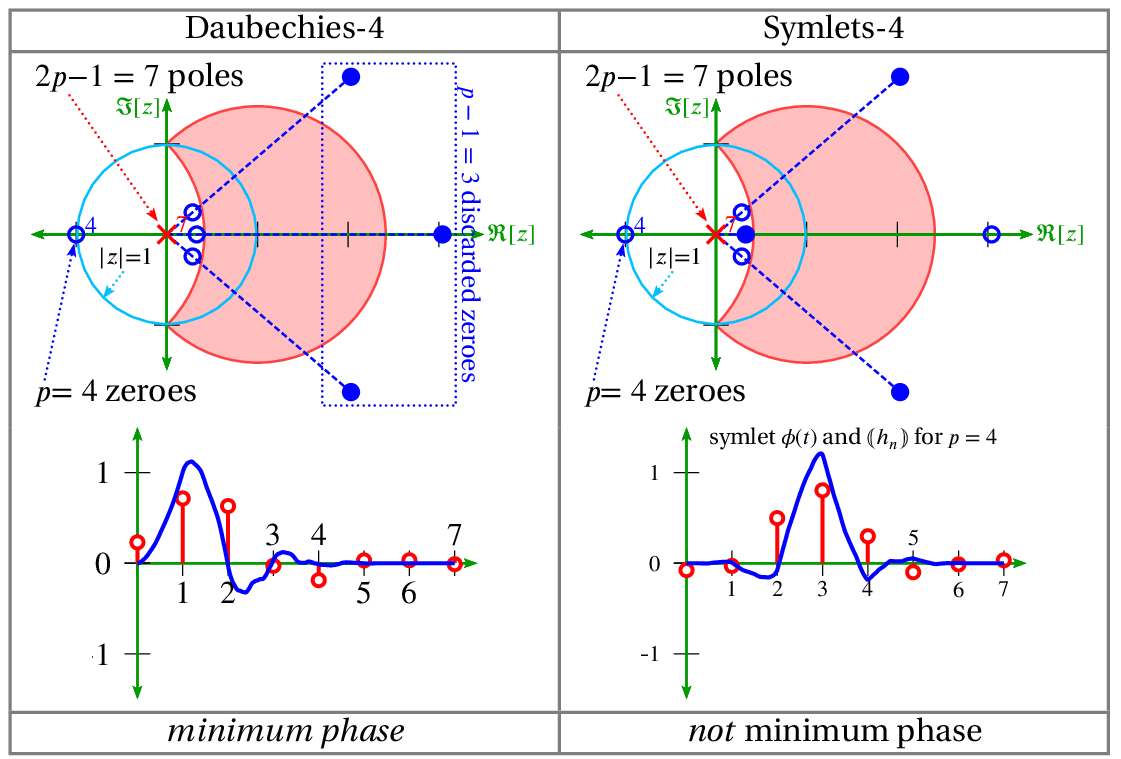# Z-Transform

June 2, 2021

The z-transform is defined as:

(1)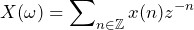### Unit Circle in Z-domain

The z-transform is a generalization of the discrete-time Fourier transform.

(2)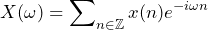Therefore, the DTFT is the unit circle portion of the z-transform.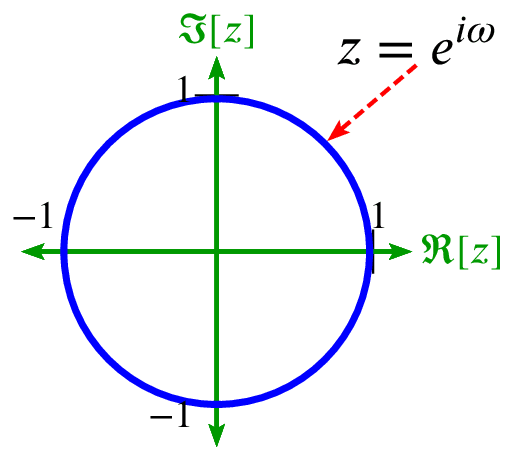The N-point DFT or FFT is N samples around the unit circle. The Fourier transform, which is a special case of the s-domain Laplace transform, can be roughly mapped into the z-plane by use of the bilinear transform.

(3)### Pole-Zero Locations

The roots of the numerator are called zeros, and the roots of the denominator are called poles.

(4)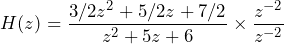(5)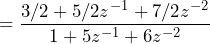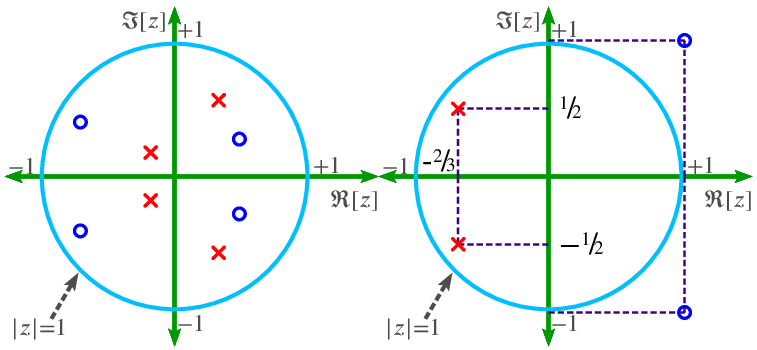### Determine Frequency Response

The frequency response of a filter is determined by the interaction of a unit vector rotating around the unit circle with the poles and zeros of the filter.

• The unit vector at rotation ω=0 corresponds to DC (0Hz).
• The unit vector at rotation ω=π (180°) corresponds to Fs/2 or the Nyquist frequency.

When the tip of the unit vector gets close to a zero, the filter magnitude response is pushed downwards because zero is a root of the numerator polynomial. When the tip of the unit vector gets close to a pole, the filter magnitude response is pushed upwards because a pole is a root of the denominator polynomial.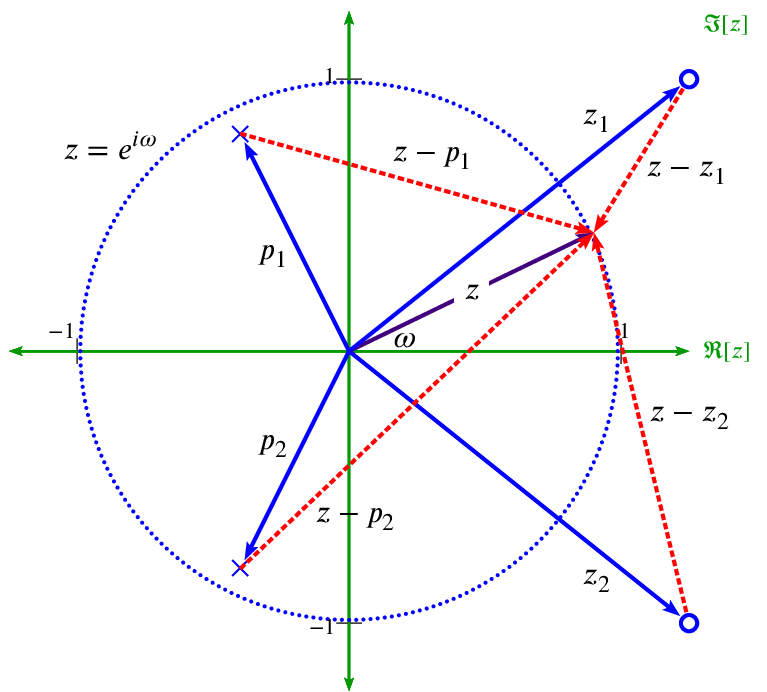Pole-zero locations are important for:

• Wavelets
• Symlets
• B-splineis